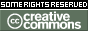Please use this identifier to cite or link to this item: `https://hdl.handle.net/10216/25790`
 Author(s): Christian LompA.J. Pena Title: A note on prime modules Issue Date: 2000 Abstract: In this note we compare some notions of primeness for modules existing in the literature. We characterize the prime left R-modules such that the left annihilator of every element is a (two-sided) ideal of R, where R is an associative ring with unity, and we prove that if M is such a left R-module then M is strongly prime. These two notions are studied by Beachy [B75]. Furthermore, if M is projectiveas left R=(0 : M)-module then M is B-prime in the sense of Bican et al. [BJKN]. On the other hand, if M is faithful then M is (strongly) prime if and only if M is strongly prime (or an SP-module) in the sense of Handelman-Lawrence [HL] if and only if M is torsionfree and R is a domain. In particular this happens if R is commutative. Description: In this note we compare some notions of primeness for modules existing in the literature. We characterize the prime left R-modules such that the left annihilator of every element is a (two-sided) ideal of R, where R is an associative ring with unity, and we prove that if M is such a left R-module then M is strongly prime. These two notions are studied by Beachy [B75]. Furthermore, if M is projectiveas left R=(0 : M)-module then M is B-prime in the sense of Bican et al. [BJKN]. On the other hand, if M is faithful then M is (strongly) prime if and only if M is strongly prime (or an SP-module) in the sense of Handelman-Lawrence [HL] if and only if M is torsionfree and R is a domain. In particular this happens if R is commutative. Subject: Álgebra, MatemáticaAlgebra, Mathematics Scientific areas: Ciências exactas e naturais::MatemáticaNatural sciences::Mathematics URI: https://repositorio-aberto.up.pt/handle/10216/25790 Document Type: Artigo em Revista Científica Internacional Rights: openAccess License: https://creativecommons.org/licenses/by-nc/4.0/ Appears in Collections: FCUP - Artigo em Revista Científica Internacional

Files in This Item:
File Description SizeFormat
49355.pdfpublished paper176.47 kBAdobe PDFThis item is licensed under a Creative Commons License